# Getting Started with WPF Surface Chart (SfSurfaceChart)

18 Oct 202220 minutes to read

This section helps you get started with `SfSurfaceChart` control.

## Visual Structure

The following image helps you understand various elements in SfSurfaceChart when you use it for the first time.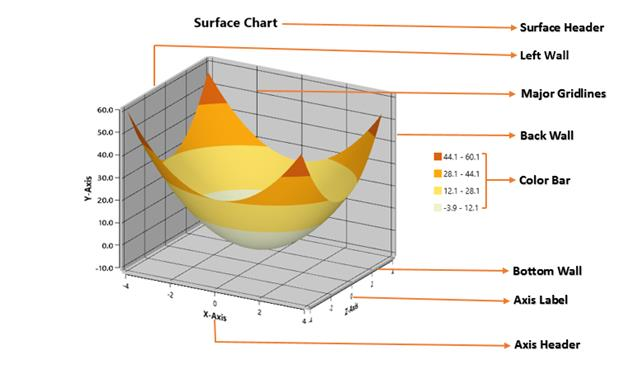• Surface Header – Represents the title of surface chart
• Wall – Represents the wall that bounds the surface chart.
• Major Gridlines- Represents the surface axis gridline for surface chart.
• Color Bar- Displays the value range in color for surface chart.
• Axis Label- Displays the label for surface axis.

## Create simple surface chart from XAML

This section demonstrates how to create a surface chart using the `SfSurfaceChart` control from XAML. You can add the `SfChart` assembly as a reference to your application to create a surface chart using SfSurfaceChart control from XAML.

2. Choose Windows > Extensions > Syncfusion.SfChart.WPF.

4. XAML
5. ``xmlns:syncfusion="clr-namespace:Syncfusion.UI.Xaml.Charts;assembly=Syncfusion.SfChart.WPF"``

NOTE

Adding the extension Syncfusion.SfChart.WPF, adds all the Syncfusion WPF controls. You can also add the SfChart reference alone from the following location. C:\Program Files (x86)\Syncfusion\Essential Studio\Assemblies (4.0/4.5.1/4.6)

### Initialize the surface chart

You need to initialize the surface chart from the following namespace Syncfusion.UI.Xaml.Charts.

``````<syncfusion:SfSurfaceChart>

</syncfusion:SfSurfaceChart>``````

## Add Surface chart from Toolbox

Drag the `SfSurfaceChart` control from the Toolbox to the required location, where the surface chart should be displayed. You can select Toolbox from the View menu, when the toolbox window is not available in the project. The Toolbox window appears on the left side of the screen.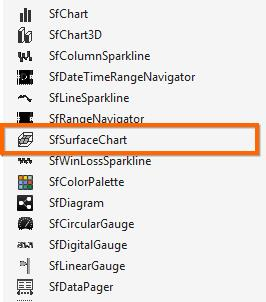The Syncfusion WPF reference is added to the application reference and the xmlns namespace is added to MainPage.xaml.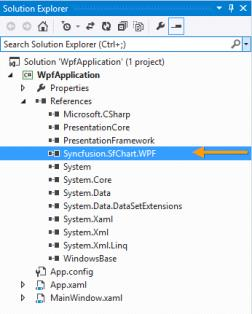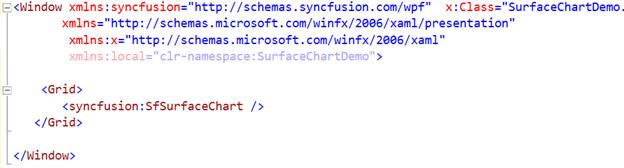The following dataset is used to plot the surface chart.

 X Y Z 0 0 0 1 1 1 2 2 2 3 2 1 2 1 2 1 2 3 0 1 2 0 1 2 0 1 2

Before proceeding with the chart, create data model with the above details as follows.

• C#
• ``````public class Data
{
public double X { get; set; }
public double Y { get; set; }
public double Z { get; set; }
}``````

Now, you have a data model property which hold values of each item in the collection.

• C#
• ``public ObservableCollection<Data> DataValues { get; set; }``

Also you have a property for row and column size in the given data.

• C#
• ``````public int RowSize { get; set; }

public int ColumnSize { get; set; }``````

Add the values to the above defined collection property called DataValues, with the values illustrated in the above table.

• C#
• ``````DataValues.Add(new Data() {X = 0, Y = 3, Z = 0});
DataValues.Add(new Data() {X = 0, Y = 2, Z = 1});
DataValues.Add(new Data() {X = 0, Y = 1, Z = 2});

DataValues.Add(new Data() { X = 1, Y = 2, Z = 0 });
DataValues.Add(new Data() { X = 1, Y = 1, Z = 1 });
DataValues.Add(new Data() { X = 1, Y = 2, Z = 2 });

DataValues.Add(new Data() { X = 2, Y = 1, Z = 0 });
DataValues.Add(new Data() { X = 2, Y = 2, Z = 1 });
DataValues.Add(new Data() { X = 2, Y = 3, Z = 2 });

RowSize = 3;
ColumnSize = 3;``````

Now you can add the elements required for the above scenario to the Surface instance created.

### Add data to surface chart

After you populate the data to the chart, you can bind the properties as shown in the following code example.

``````<syncfusion:SfSurfaceChart ItemsSource="{Binding DataValues}"  XBindingPath="X"

YBindingPath="Y" ZBindingPath="Z"

RowSize="{Binding RowSize}"

ColumnSize="{Binding ColumnSize}" >``````

The header acts as the title of the surface chart created, to identify its purpose.

``<syncfusion:SfSurfaceChart Header="Simple Surface" FontSize="20" />``

### Add Axes to surface chart

The following code example illustrates how to add XAxis, YAxis and ZAxis to the Surface chart.

``````<syncfusion:SfSurfaceChart ItemsSource="{Binding DataValues}"  XBindingPath="X"
YBindingPath="Y" ZBindingPath="Z" RowSize="{Binding RowSize}"
ColumnSize="{Binding ColumnSize}">

<syncfusion:SfSurfaceChart.XAxis>

</syncfusion:SfSurfaceChart.XAxis>

<syncfusion:SfSurfaceChart.YAxis>

</syncfusion:SfSurfaceChart.YAxis>

<syncfusion:SfSurfaceChart.ZAxis>

</syncfusion:SfSurfaceChart.ZAxis>

</syncfusion:SfSurfaceChart>``````

NOTE

SfSurfaceChart supports default axes, all the axes are generated automatically, even when it has not been defined explicitly.

The following code example illustrates how to add surface type to the surface chart.

``<syncfusion:SfSurfaceChart Type="Surface" />``

### Add Color bar to the surface chart

The following code example illustrates how to add color bar to the surface chart

``````<syncfusion:SfSurfaceChart.ColorBar>
<syncfusion:ChartColorBar ShowLabel="True" DockPosition="Right"/>
</syncfusion:SfSurfaceChart.ColorBar>``````

Now you have created a simple surface chart. The following code example is for XAML.

• XAML
• ``````<syncfusion:SfSurfaceChart Type="Surface"  Tilt="15" Rotate="30"

ItemsSource="{Binding DataValues}"  XBindingPath="X"

YBindingPath="Y" ZBindingPath="Z"

RowSize="{Binding RowSize}"

ColumnSize="{Binding ColumnSize}">

<syncfusion:SfSurfaceChart.XAxis>

</syncfusion:SfSurfaceChart.XAxis>

<syncfusion:SfSurfaceChart.YAxis>

</syncfusion:SfSurfaceChart.YAxis>

<syncfusion:SfSurfaceChart.ZAxis>

</syncfusion:SfSurfaceChart.ZAxis>

<syncfusion:SfSurfaceChart.ColorBar>

<syncfusion:ChartColorBar  DockPosition="Right"/>

</syncfusion:SfSurfaceChart.ColorBar>

</syncfusion:SfSurfaceChart>``````

The following output is displayed as a result of the above code example.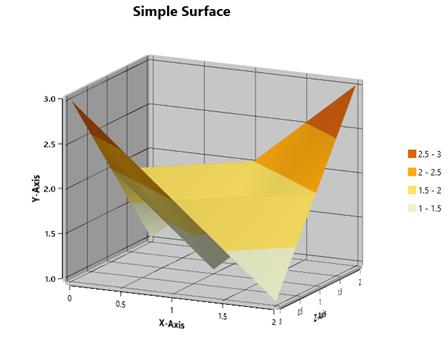## Create a simple surface chart from Code behind.

This section demonstrates how to create a surface chart using SfSurfaceChart control from code behind. For that, you can add the SfChart assembly reference to your application.

2. Choose Windows > Extensions >Syncfusion.SfChart.WPF.
3. Add the following namespace in code behind

4. C#
5. ``using Syncfusion.UI.Xaml.Charts;``

The following section demonstrates a simple surface chart with the data discussed in the above table.

### Initialize the surface chart

• C#
• ``SfSurfaceChart surface = new SfSurfaceChart();``

### Add Data to surface chart

You can set the surface chart data in code behind, by directly adding data points to the Data property of surface chart using AddPoints(x,y,z) method as shown in the following code example.

• C#
• ``````SfSurfaceChart surface = new SfSurfaceChart();

//First Row

//Second Row

//Third Row

surface.RowSize = 3;
surface.ColumnSize = 3;

The header acts as the title of the surface chart created, to identify its purpose.

• C#
• ``````SfSurfaceChart chart = new SfSurfaceChart();
chart.FontSize = 20;

### Add Axes to surface chart

The following code example illustrates how to add XAxis, YAxis and ZAxis to the surface chart.

• C#
• ``````SfSurfaceChart chart = new SfSurfaceChart();
chart.SetBinding(SfSurfaceChart.ItemsSourceProperty, "DataValues");
chart.SetBinding(SfSurfaceChart.RowSizeProperty, "RowSize");
chart.SetBinding(SfSurfaceChart.ColumnSizeProperty, "ColumnSize");
chart.XBindingPath = "X";
chart.YBindingPath = "Y";
chart.ZBindingPath = "Z";

SurfaceAxis xAxis = new SurfaceAxis();
chart.XAxis = xAxis;

SurfaceAxis yAxis = new SurfaceAxis();
yAxis.LabelFormat = "0.0";
chart.YAxis = yAxis;

SurfaceAxis zAxis = new SurfaceAxis();
chart.ZAxis = zAxis;

NOTE

SfSurfaceChart supports default axes, where all the axes are generated automatically, even when they are not defined explicitly.

The following code example illustrates how to add surface type to the surface chart.

• C#
• ``````SfSurfaceChart chart = new SfSurfaceChart();
chart.Type = SurfaceType.Surface;

### Add ColorBar to the surface chart

The following code example illustrates how to add color bar to the surface chart.

• C#
• ``````ChartColorBar colorBar = new ChartColorBar();
colorBar.DockPosition = ChartDock.Right;
colorBar.ShowLabel = true;
chart.ColorBar = colorBar;``````

Now, you have created a simple surface chart. The following code example is for code behind and data can be populated either by using the`ItemsSource` property or by using the `AddPoints` method of `Data` property in surface chart.

• C#
• ``````SfSurfaceChart chart = new SfSurfaceChart();
chart.SetBinding(SfSurfaceChart.ItemsSourceProperty, "DataValues");
chart.SetBinding(SfSurfaceChart.RowSizeProperty, "RowSize");
chart.SetBinding(SfSurfaceChart.ColumnSizeProperty, "ColumnSize");
chart.XBindingPath = "X";
chart.YBindingPath = "Y";
chart.ZBindingPath = "Z";

or

SfSurfaceChart surface = new SfSurfaceChart();
surface.Tilt = 15;
surface.Rotate = 30;
surface.Type = SurfaceType.Surface;

//First Row

//Second Row

//Third Row

surface.RowSize = 3;
surface.ColumnSize = 3;

SurfaceAxis xAxis = new SurfaceAxis();
surface.XAxis = xAxis;

SurfaceAxis yAxis = new SurfaceAxis();
surface.YAxis = yAxis;

SurfaceAxis zAxis = new SurfaceAxis();
You can get the complete getting started sample `here`.